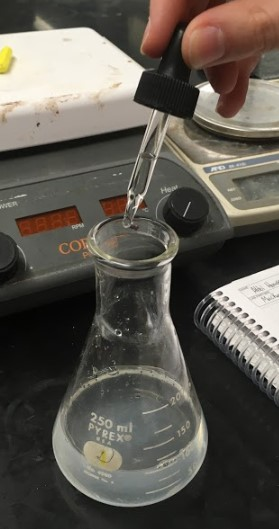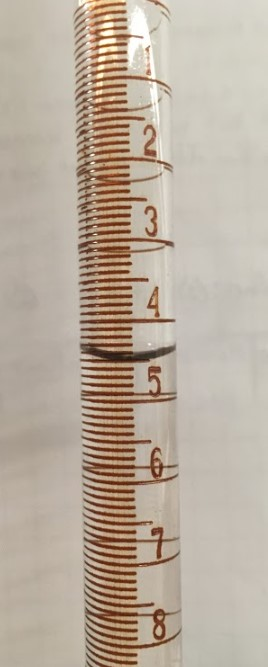# 7: Titration of Fruit Juices

Objectives

• To determine the amount of citric acid present in fruit juices.

Some citrus fruits taste more sour, and therefore are more acidic, than others. It is the purpose of this experiment to determine the total acidity of orange, lemon, grapefruit, white grape or lime juice. This procedure can be used to determine the total acidity of most natural fruit juices without further preparation.

Citric acid is the acid primarily responsible for the sour taste in citrus fruits. Citric acid is a polyprotic acid in which three ionizable hydrogens may be neutralized by a strong base.

Pre-Lab Assignment

• Look up the Lewis structure of citric acid and draw it in your laboratory notebook. Remember to cite your source.
• On your structure, circle the ionizable hydrogens for each acid group.
• Write a balanced reaction of the titration of citric acid with NaOH.

## Procedure

Safety and Waste Disposal

• The solutions can be disposed of down the drain.

###### Step 1Using your 10 mL graduated cylinder, measure out 5.00 mL of juice into the flask.

###### Step 2

If the juice is highly colored, measure a smaller volume of (1.00 - 2.00 mL) sample.

###### Step 3Dilute the juice to approximately 100 mL with distilled water.

###### Step 4Add 5 drops of phenolphthalein indicator.

###### Step 5Record the initial volume of standardized NaOH solution obtained in last week’s experiment to the nearest 0.01 mL.

###### Step 6

Titrate the prepared juice solution to the phenolphthalein endpoint.

###### Step 7

With colored juice, the endpoint is not as clear and may occur over 3-4 drops of titrant.

###### Step 8Record the final burette reading to the nearest 0.01 ml.

###### Step 9

Repeat the experiment at least once or until the percent difference is within 5%.

###### Step 10

Obtain a sample of a different juice and perform at least 2 titration trials until they agree within 5%.

## Calculations

### First Fruit Juice – Juice Identity __________________

Data Trial 1 Trial 2 Trial 3 (Trial 4)*
Volume of Juice
Initial $$\ce{NaOH}$$ buret reading:
Final $$\ce{NaOH}$$ buret reading:
Volume of $$\ce{NaOH}$$ used:

###### Step 1

Calculate the volume of $$\ce{NaOH}$$.

###### Step 2

Calculate the moles of $$\ce{NaOH}$$.

###### Step 3

Calculate the number of moles of citric acid.

###### Step 4

Calculate the mg of citric acid.

###### Step 5

Calculate the mg of citric acid per mL of juice.

###### Step 4

Determine if your result of the concentration for citric acid after two titrations is within the tolerance. Calculate the percent difference of two titrations.

$\%\: difference = \frac{|M_{1} - M_{2}|}{M_{2}} \times 100 \label{4}$

Calculations Trial 1Trial 1Trial 1Trial 1 Trial 2 Trial 3 (Trial 4)*
Moles of $$\ce{NaOH}$$ used:
Moles of citric acid titrated:
mg of citric acid:
mg of citric acid/mL of juice:

*If necessary

• Average molarity of $$\ce{NaOH}$$ solution from week one: ___________________ M

In the space below, clearly show all calculations for your Trial 1 data only: